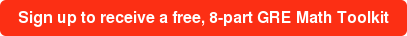What does the fox say? Expo-expo-expo-exponents!

I'm going to make a bold claim: the trickiest, most fiendish section you will find on any American standardized test isn't the logic games of the LSAT or the physical sciences part of MCAT: it's the entire GRE quantitative reasoning test. Why? Because the makers of the test can only test the same knowledge that was on the SAT, but they know that almost every student has had at least three extra years to practice those math skills. And so, for those who have more or less blown off math since they started college (humanities majors, I'm looking at you), the quantitative section of the GRE is absolutely terrifying.

Beyond the mechanics of math, the GRE also asks questions in funny ways and hides answers. The writers of the GRE can manipulate a mathematical statement in a dizzying number of ways, and they might require that you do the same thing. In this blog post, I’ll teach you how to recognize and solve a type of problem that GRE test writers commonly use to trick students: exponents.

#### The following problem could be taken directly from a GRE. It’s a quantitative comparison question, asking whether Quantity A or Quantity B is bigger, or are they equal? Because the question deals with real numbers, we can eliminate option D (Can’t tell).

 Quantity A Quantity B 164 216

To solve this problem, I’ll (surprisingly for anyone who knows me) turn to fashion. I lack any sense of it. This disability leads to situations where I am extremely underdressed, or extremely overdressed. Simply put, I don’t have any idea what kind of target to aim for. The correlation between occasion and outfit eludes me and so I don’t know how to look for an occasion. In my years as a GRE tutor, I've learned that students that struggle with GRE math may not recognize it, but they suffer from the same dilemma, but in a slightly different form. Instead of making sure that the clothes fit the occasion, a test-taker needs to make sure that the numbers fit the answer choices.

At first, quantity A and Quantity B look very different. But, by massaging one of the quantities to look like the other one, this problem becomes solvable. A good GRE test taker realizes that a good strategy is to simply make her numbers look like the GRE’s numbers.

Practically, this means fiddling around with numbers. Because it’s an exponent question, I would guess that the GRE wants to test my knowledge of exponents. With the knowledge that I want to make Quantity A look like Quantity B, I start tinkering:

1. My end goal is to rewrite 164 using only 2’s and exponents.

2. 164 could be rewritten as (42)4 or 48.

3. If I apply that process of rewriting again, I get 4=22 so 48= (22)8.

4. Applying exponent rules, I see that (22)8, is equal to quantity B.

5. Therefore, the answer is C: the two values are equal.

#### Math needs some kind of target. Let the answer choices on a standardized test guide you towards the right answer or what the right answer should look like.Tags: GRE, math